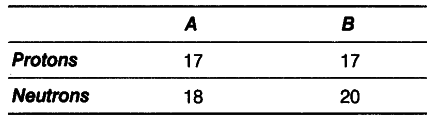# What are the mass numbers of A and B?

Composition of the nuclei of two atomic species A and B are given below.(i) What are the mass numbers of A and B?
(ii) How are they related to each other? Define the term.

(i) Mass number of A = Number of protons + number of neutrons = 17 + 18=35
Mass number of B = 17 + 20 = 37
(ii) They are isotopes of same element. Isotopes are the atoms of same element having the same atomic number but different mass numbers.